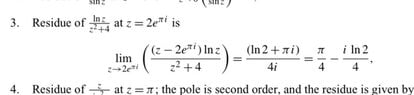# Calculating Residue

curious_mind
Homework Statement:
Calculate the residue of the complex function ## f(z) = \dfrac{\ln z}{z^2 + 4} ## at ## z = 2 e^{i \pi} ##
Relevant Equations:
## a = \lim_{z \rightarrow z_0} \left[ \left(z_0 - z\right) f(z) \right] ##
This question is given as an example in the book by
Arfken, Weber, Harris, Mathematical Methods - a Comprehensive Guide, Seventh Edition.

It is solved as below attached in the image.

Can someone point it out how they proceed with calculations ? I do not seem to get their calculation.
I am aware ## \ln z ## is a multivalued function. But at this point I do not know things about Branch points and etc.

According to my understanding the function is not singular at point ## z=2 e^{i \pi} =−2 ## . So why they have used limits ?

Thanks.

#### Attachments

•20230317_074323.jpg
13.4 KB · Views: 4
•vanhees71

Mentor
2022 Award
•vanhees71 and curious_mind
curious_mind
Even then, in numerator, it should be ## \ln 2 + i \dfrac{\pi}{2} ## right ?

•vanhees71
Staff Emeritus
Homework Helper
I guess there is a typo and it should be ##z_1=2e^{i \pi /2}.##
Or the denominator was supposed to be ##z^2-4##. In either case, the example is all messed up.

Mentor
2022 Award
Even then, in numerator, it should be ## \ln 2 + i \dfrac{\pi}{2} ## right ?
Yes, ...
Or the denominator was supposed to be ##z^2-4##. In either case, the example is all messed up.
... and yes.

At least, ##2e^{i \pi /2}## is a singularity. However, ##z=-2## would be faster to solve.

•SammyS